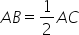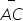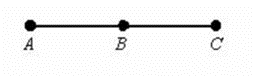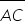Mathematics
Easy

Question

# What is the missing statement in the proof?Given:Prove: B is the midpoint ofStatements ReasonGiven 2AB = AC Multiplication property of equality AB + AB = AC Distributive property AB + BC = AC ______? AB + AB = AB + BC Transitive property of equality AB = BC Subtraction property of equality B is the midpoint ofDefinition of midpointHint:

### if a is any point on the line xy thenxa + ay = xy . This is Segment Addition Postulate  .

The segment addition postulate in geometry is the axiom which states that a line segment divided into smaller pieces is the sum of the lengths of all those smaller segments. So, if we have three collinear points A, B, and C on segment AC, it means AB + BC = AC. It is a mathematical fact that can be accepted without proof.

### Related Questions to study#### With Turito Foundation.#### Get an Expert Advice From Turito.